## Sunday, September 30, 2007

Hi, I'm Roslyn. Sorry if i posted up my scribe post late, I've been very busy. But yeah on Friday morning, Ms. Nickelson (Sorry if i cant spell the last name correctly =/ ), explained to us the stretches and reflections of a quadratic formula.

Stretches :
ex. f(x)= 2sinx <-- stretches vertically any number greater than 1 stretches vertically. ex. f(x) = (1/2x) <-- stretches horizontally any number greater than 1 compresses horizontally. Reflections:
If there is a negative sign in front of the formula,
ex. y= - f(x)
then the graph flips over the x-axis.

If there is a negative sign inside the brackets,
ex. y = f(-x)
then the graph flips over the y - axis.

And yeah thats pretty much what we did last class. And we were assigned exercise #8.

Tomorrow's scribe will be ALANNA.

## Thursday, September 27, 2007

### Stretches & Compressions, A Video Lesson

A student emailed me who was having trouble understanding this lesson. I recorded this video minutes afterwards. It took me a while to compress it and get it published online. This was the first time I've ever done this. I learned a few things I would do differently to improve it next time. Your feedback is welcome and encouraged. ;-)

The video is 16 minutes and 20 seconds long. After watching this you should be able to do almost all the questions in exercises #8 and #9.

Two things: For some reason the video is about 12 seconds ahead of the audio. Also, at one point I misspoke and described y=f(-x) as a reflection over the x-axis. That's not right, it's a reflection over the y-axis. Everything else I said about that transformation is correct.

Here's the Google video ...

A lot of the video quality was lost. There's a better (not best) version here. Click that link to see a much better quality video.

A nice thing about video ... you can [pause] [rewind] [repeat] as often as you like until you "get it." Nonetheless, feel free to ask questions ... in the comments to this post or when I see you on Monday.

You may also find these links helpful ...All about vertical reflectionsAnd you can find a tutorial and interactive java applet here to review and learn all about vertical and horizontal stretches, compressions and reflections.

Let me know which link you liked the best. ;-)

### REVIEW + TEST DAY

Hey everybody, it's Ivanna. Today was an easy class.

For our first period class, we had a work period to study a review Ms. Nicholson gave us.
For our second period class, we all wrote our very first test, "Circular Functions".

Tomorrow's scribe will be ... ROSLYN

## Wednesday, September 26, 2007

### Transformations

Hello all, this is Lina with today's scribe post.

This morning we went over the last question on our pre-test from yesterday. Miller has the answers to the questions from the pre-test in his post yesterday. We also had a practice question where we had to draw out a graph and write a second equation for it. That can be seen in the first slide post for today. In the first post of "Today's Slides" are questions to help us review for the test tomorrow during fourth period. Don't forget to do them! We were also asked to complete Exercise 7.

After all that jazz, we went on to start our new unit, "TRANSFORMATIONS". How exciting.
A simple definition of would be that: transformations are operations that change or alter the way that a function looks.
The type of transformation that we've learned today was Translation. A definition of a translation would be that it changes the position of the function without modifying its size or orientation. Basically it would shift the function to a different point on the graph.
The general equation for this would be ----> y= f(x - a) + b
In the equation, a would move the function left or right where b would move the function up or down.

For example, we were given this question in class today:
The graph of g(x) is formed by sliding f(x) 4 units to the left. If f(x) = sin(x-2) + 5, write the equation in terms of sine to represent g(x).

The graph for f(x) would look like this: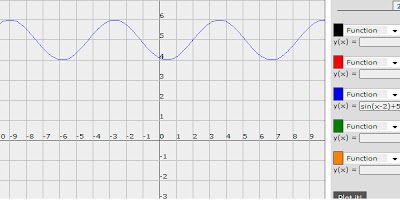Now, the graph for g(x) is moving 4 units to the left. We know the general equation is y= f(x-a)+ b and we know it's a that has to change because it's the one that moves the function left or right.

If f(x)= sin(x-2)+5. Then g(x)= sin(x+2) + 5 so that the function shifts 4 units to the right as shown in green in this next graph.You can count it if you like, but I assure you it has shifted 4 units.

Using the same equation of : f(x)=sin(x-2)+5, we can also shift the function up or down by changing the b value. If we wanted to say, find g(x) by shifting the f(x) down by 3 units, the equation would look like: g(x)=sin(x-2)+2. f(x) is shown in blue, g(x) is shown in green.Pretty easy right? So let's conclude. Translation is when we shift the function to a different point on the graph without changing its size or orientation. The general equation for the function is: y=(x-a)+b. While using translation, the only values we would change would be the a and b values.

With that said, I will now end my post. I hope I did our new unit justice and I hope you all understand what I was trying to explain.

REMEMBER! Our test is tomorrow, fourth period! So study, study!

Last, but not least, the next scribe will be... GOAT.

### Today's Slides: September 26

Here they are ...

To see a larger image of the slides go here. When you get there you'll see a button in the bottom right-hand corner that says [full]. Click it and the slides will display in full screen mode.

### Today's Slides: September 26

Here they are ...

To see a larger image of the slides go here. When you get there you'll see a button in the bottom right-hand corner that says [full]. Click it and the slides will display in full screen mode.

## Tuesday, September 25, 2007

### Circular Functions Pre Test

Good Evening, this is Miller reporting today on our blog. Unfortunately our beloved teacher Mr. K was not in class today. Instead we had the charming Miss Nicholson. During our first period class we had a quiz yet again. I can't remember what was on the quiz since we handed it in afterwards. Once we were done the quiz we were allowed to study for the afternoon pretest. The test looked like this: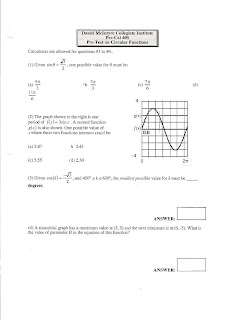Here are the answers we received in class today: (n) = pie ( I couldn't find a symbol for it)
(€)= theta
1) b) 2n/3, because sin€ is only equal to √3/2 in the first and second quads.

2) b) 2.41, y=2
f(x)=3sin(x)
2=3sin(x)
sinx=2/3

3) It asks for cos(k)= -√3/2 between the intervals of 450 degrees and 630. 5n/6 is what cos is when it is at -√3/2. The measure of that angle is 150 degrees. This means the have to add 360 to it in order for it to be in the correct intervals. The answer is 510 degrees.

4) Here we are looking for the B of the equation. AsinB(x-C)+D. We find out the period of the graph and then use the equation B=2n/period.
B=2n/8
= n/8
5) A) Here we are asked to find the period of the function p(t)=100-20cos5nt/3. I arranged it so it would look like p(t)= -20cos5n/3+100. We take the B of this equation and use it to divide 2n.
Period=2n/B
2n/1 * 3/5n
= 6/5
B) Our sinusoidal axes is now at 100. Since the A of the function is -20 it means that it will go 20 below 100 and also 20 above 100.
Max= 120
Min= 80

c) This is what the graph of the cos should look like:Tommorow I believe we are going to do something new if Mr. K is there. Reminder that our test is on Thursday. The next lucky blogger is Lina.

## Monday, September 24, 2007

### Monday, September 24, 2007

Hello, my name is Kimberley and I am the scribe for today.

We started off the class today by doing a short quiz. It was out of 16 and we marked it in class. If you missed it for some reason they are in the slides posted up by Mr. Kuropatwa. After the quiz we were put into groups to work on a problem. We were asked to come up with 2 equations for a graph. One being sine and the other cosine. We didn't quite finish discussing it, but we did come up with the sine equation. Here is the slide:

The answer might be kind of hard to read, but this is what it is: Y = 2sin3(X-π/6) +1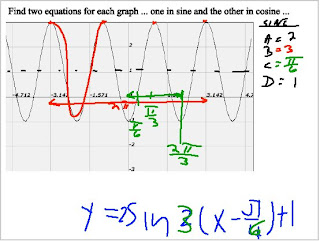In tomorrow's class we will be having a pre-test on Circular Functions. Then on Wednesday we will be starting our new unit :Transformations.

*REMINDER: our unit test on Circular Functions will be on Thursday, September 27, 2007. Don't forget to study.

Tomorrow's scribe is MILLER

### Today's Slides: September 24

Here they are ...

To see a larger image of the slides go here. When you get there you'll see a button in the bottom right-hand corner that says [full]. Click it and the slides will display in full screen mode.

## Sunday, September 23, 2007

### circular functions

hi its Anthony. Sorry i started this so late, i was at work for the last few days. Anywho we started off the class doing some warm up graphing questions. later in the class Mr. K started something new. Writing equations for graphs. He started off by showing the relationship between cos(x) and sin(x). Turns out both of these graphs are the same, only difference is the horizontal shift. What i mean by that is:cos (x) = sin(x+π/2) and sin(x) = cos(x-π/2)

Now moving on. Graphing graphs from equations is easy but writing equations from graphs was something new to most of us on friday. Remember when writing equations for graphs use DABC. What i mean by DABC is find the verticle shift, amplitude, period, and the horizontal shift. Also Mr. K can never stress enough that i quote " B IS NOT THE PERIOD... B IS NOT NOT NOT NOT NOT THE PEIORD." B helps determin the period. with the following equation:

period = 2π/B

Another new thing we learned was how to graph tan(x).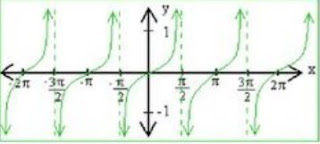the dotted lines are the asymptotes (where the graph will never touch). the reason they will never touch is because on the unit circle at those specific points because tan is parallel with the y axis. lastly graphing trig functions should be in your math dictionarys. The next scribe is

Kim-Possible.

## Friday, September 21, 2007

### Today's Slides: September 21

Here they are ...

To see a larger image of the slides go here. When you get there you'll see a button in the bottom right-hand corner that says [full]. Click it and the slides will display in full screen mode.

## Thursday, September 20, 2007

### CIRCULAR FUNCTIONS

Hello fellow classmates,
I am SAM and I'll be your scribe for the day. First thing we did was answer Mr. K's question. He asked us individually if we had read his post, Students Made This. Fortunately enough, everyone did. So expect a chat box and a walkie-talkie device thingy soon. Right after that we jumped right into math. We started off with the equation y= Cos2x, which we had to graph.STEP 1:
check if parameter D is present.

STEP 2: check if parameter A is present.
STEP 3: check if parameter B is present. Since B is 2 that means that the period has changed. and to find the period you divide 2π/ B. So 2π/ 2 = π, the period is π. That means that there are now two waves between 0 and 2π.
STEP 4: check if parameter C is present.
STEP 5: Now that you know it's a cos graph and you know what the period is, you're ready to sketch the graph.The second graph we had to sketch was y=sin(x+π/4)A useful Mnemonic would be DABC!

STEP 1: check if parameter D is present.
STEP 2: check if parameter A is present.
STEP 3: check if parameter B is present.
STEP 4: check if parameter C is present. Since C is the phase shift or horizontal shift and the value of C is +π/4, that means that the graph will shift to the left π/4 radians. It shifts to the left because in the original equation C is negative.
STEP 5: Now you're ready to sketch the graph. you know that it's a sin graph and it shifts horizontally to the left π/4 radians.The next equation we had to graph was y=cos2(x-π/4).

STEP 1: check if parameter D is present.
STEP 2: check if parameter A is present.
STEP 3: check if parameter B is present. Since B is 2 that means that the period has changed. and to find the period you divide 2π/ B. So 2π/ 2 = π, the period is π. That means that there are now two waves between 0 and 2π.
STEP 4: check if parameter C is present.Since parameter C is the horizontal shift and the value of C is -π/4, that means the graph will shift to the right π/4 radians. It shifts to the right because in the original equation the value of C is negative.
It's like saying y=AcosB[x-(-C)]+ D. The negatives cancel.
STEP 5: Now that you know it's a cos graph and you know what the period and shift is, you're ready to sketch the graph.We had to graph an equation with all the parameters, which was
y= -1/2sin3(x+π/6)+2.
REMEMBER: DABC!

STEP 1: D, the value of D is +2, so that means the sinusoidal axis is going to be shifted up +2. The sin graph will wind around this line.STEP 2: check if parameter A is present. Since the value of parameter A < color="#000000">And since A
is negative that means it will be flipped. where the max's are they will now be min and where the min are they will be max's.STEP 3: check if parameter B is present. Since B is 3 that means that the period has changed. and to find the period you divide 2π/ B. So 2π/ 3 = 2π/3, the period is 2π/3. That means that there are now three waves between 0 and 2π.STEP 4: check if parameter C is present.Since parameter C is the horizontal shift and the value of C is π/6, that means the graph will shift to the right π/6 radians. It shifts to the right because in the original equation the value of C is negative.
It's like saying y=AcosB[x-(+C)]+ D. The value C stays negative.

STEP 5: Now that you know it's a cos graph and you know what the vertical shift, stretch, period, and what the phase shift is you're ready to sketch the graph.Here's a link to see how the unit circle is related to the sin graph CLICK HERE!

And that summarizes today's math class.
Tomorrow, our scribe for the day will be
.....................................................................................................
.....................................................................................................
.....................................................................................................
.....................................................................................................
.....................................................................................................
.....................................................................................................
ANTHONY

### Today's Slides: September 20

Here they are ...

To see a larger image of the slides go here. When you get there you'll see a button in the bottom right-hand corner that says [full]. Click it and the slides will display in full screen mode.

## Wednesday, September 19, 2007

### Circular Functions

Hey guys, sorry for the really late post. Today, we started class by answering these three questions: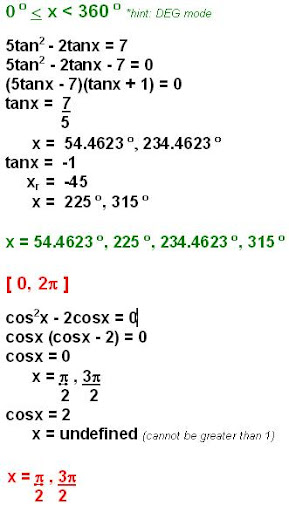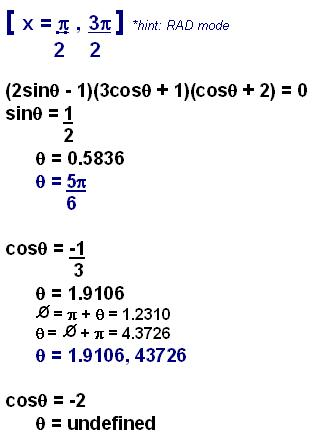For the second half of class we had mental math. We then learned how to plot sine and cosine functions onto a graph which pretty much took the whole period.
The basic sine function: y = sin (x). Even though the sine and cosine graph looks the same, they have different starting points. But, this could easy be changed if cosine was to be shifted either to the left or right by half of pi.

ex.)
3 sin 4(x - 8) + 5
the distance between the min/max value from the average value
compresses/stretches the graph
x-value shifts left/right; if negative, shifts right. if positive, shifts left
y-value shifts upwards/downwards; if negative, shifts downwards. if positive, shifts up.

Tomorrow, we will continue with what we learned today, but Mr. K will further explain the function of cosine. Sorry, if this wasn't much help. I don't really understand the whole function graph thing yet, but Mr. K will continue where we left off today so it's all good. Oh and sorry i didn't post up a graph to show my function of sine.

HOMEWORK: one slide down :] questions (a) through (f) ; sketch graph.

The next blogger is SAMUS

### Graph Trig Functions Homework

Here is the homework for tonight. Do only questions (a) through (f). The rest is for homework tomorrow night. ;-) After you have sketched each graph without using your calculator, you can use your calculator or fooplot.com to check your work.

Cheers!
Mr. K.### Today's Slides: September 19

Here they are ...

To see a larger image of the slides go here. When you get there you'll see a button in the bottom right-hand corner that says [full]. Click it and the slides will display in full screen mode.

## Tuesday, September 18, 2007

### Circular function...

Our class today started with these things. Its somewhat a review and a new lesson i think.

Then after that, we did a mental math about the radian things.
Then we answered the following question. Where mr. k told us about the real number things which is related to our homework located at the bottom of the picture.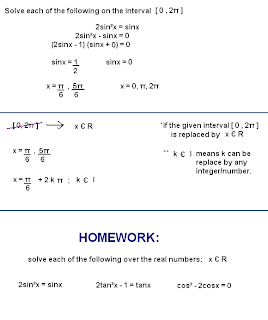Well anyways. i think i covered everything. nd I think some or more will be confused about my post because i didnt really explained everything thoroughly. Its just that its hard for me too to expalin it. And I kinda dont want to write long things. It just wont seem like the summary of what we did today it'll be more of an essay of some sort. Well thats probably it, and i hope my post help some people even though its soo late and too simple. and uhmm HOMEWORK is EX. 5 all of it. 1-20 i mean. and the next blogger will be MICHELLE S.

### Today's Slides: September 18

Here they are ...

To see a larger image of the slides go here. When you get there you'll see a button in the bottom right-hand corner that says [full]. Click it and the slides will display in full screen mode.

## Monday, September 17, 2007

### Unit Circle

Hello! Today, as usual, Mr. K gave us questions to answer. Here they are:Then, we did a little mental math after that.

For the afternoon class, we started off with mental math, again. Btw. make sure that you also memorized not only sine and cosine values but also tangent values! He shared some of his memory tricks. Mr. K says that we are having mental math everyday this week! Again, he gave us questions:The next set of questions are similar but not the same. For the next set of questions, you may see the same numbers. However, for those questions, you must find the value of either sine, cosine, or tangent.Remeber to always write your answers IN RADIANS! not degree!
Uhm... i think that pretty much covered the whole two classes we had today? Mr. K just basically explained again about how tangent = sine over cosine.

For homework, make sure that you have exercise 4 # 1 - 10 finished for tomorrow.
If i missed anything or made a mistake, i'm really sorry, and feel free to comment and inform me =) please and thank you!!! And remember, we have mental math tomorrow, so again, make sure you know COSINE, SINE, AND TANGENT VALUES OF THE UNIT CIRCLE!!

Anyways, to conclude... the next scribe is ... REMYSHIRE =)

### Today's Slides: September 17

Here they are ...

To see a larger image of the slides go here. When you get there you'll see a button in the bottom right-hand corner that says [full]. Click it and the slides will display in full screen mode.

### The Scribe List

This is The Scribe List. Every possible scribe in our class is listed here. This list will be updated every day. If you see someone's name crossed off on this list then you CANNOT choose them as the scribe for the next class.

This post can be quickly accesed from the [Links] list over there on the right hand sidebar. Check here before you choose a scribe for tomorrow's class when it is your turn to do so.

Cycle 2
 GOATd3r3kJasminMeeeelaalannaAlvin G.(-1)AnthonySAMUSEcko MontanaSergio JoeShaiyanJessiccaIle joséphkimberleychrycellinaLuisoliver_796 Pauloprecious_01'25'26RemyshirerosselleSharmaineD.michellewendy(-1) Mary Annxxcool-guyxxcheyyt.(-1)

## Friday, September 14, 2007

### Circular Functions

Hey people! This is JessiccaI as scribe for today's class. It was another day without Mr. K, but he didn't leave us to not do any work. The substitute split us into groups of two to three and each group was handed a sheet of paper that had a question which we would answer then hand in. We were also told to hand in the Working with the Unit Circle sheet to the substitute, if not today, then at Monday.

The next scribe is CHRYCEL!!!

## Thursday, September 13, 2007

### Unit Circle

Hey guys!(: To start off my post, Mr. K was away today but as usual there's a substitute teacher. We had our first quiz on Circular Functions. After we finished the quiz, the sub handed out a worksheet called "Working with the Unit Circle". Together with the worksheet, we were also asked to do Exercise 5, questions 11-20.

Don't forget to finish the worksheet and hand it in tomorrow morning! (:

Next scribe is Jessica!

## Wednesday, September 12, 2007

### Circular Functions Assigments

For those of you that don't yet have all the exercises and solutions for our first unit on Circular Functions, here they are ...

Exercises #1 and #2 can be found here.

Exercise #3: Questions and Solutions

Exercise #4: Questions and Solutions

Exercise #5: Questions and Solutions

Exercise #6: Questions and Solutions

### -...-

hi!Unfortunatley Mr. K is absent for today so we're just asked to answer exercise 4.... that's all... and be ready for a mental math tomorrow....c yah ^-^

## Tuesday, September 11, 2007

### Unit Circle!

Hey folks sorry for waiting, i`m a having a really bad technical error today. Anyways today's class started with Mr. K asking each and everyone of us if we read the post about 'Student Made This'. Then he gave us four questions to answer.

1. In which quadrant is P(5) found?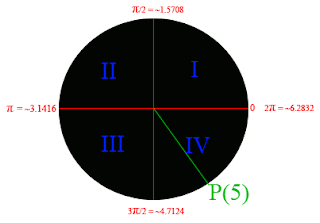Quadrant IV

->P(5) lies on quadrant IV beacause
QI is between 0-~1.5708
QII is between ~1.5708 - ~ 3.1416
QIII is between ~3.1416 - ~~4.7124
QIV is between ~4.7124 - ~6.2832

2. State a positive coterminal angel for -π/3?-> 5π/3 is a positive coterminal angle of -π/3
coterminal angle = given angle ± 2π
(-π/3 )+ 2π = 5π/3
5π/3 and -π/3 has the same initial and terminal side.

3. In which quadrant is
sin θ <> 0?

Quadrant IV

Quadrant I sin θ > 0 and cos θ > 0

Quadrant II sin θ > 0 and cos θ <>

Quadrant III sin θ <>

Quadrant IV sin θ <> o

4. In which quadrant is
csc θ <> 0?

Quadrant IV

Quadrant I csc θ > 0 and sec θ > 0

Quadrant II csc θ > 0 and sec θ <>

Quadrant III csc θ <0>

Quadrant IV csc θ <> o

After doing those problems, Mr. K introduced to us the other 3 trigonometric functions. So we now know 6 trig functions: sine, cosine, tangent, cosecant, secant, and cotangent.

sin θ = opp/hyp.............................csc θ = hyp/opp

cos θ = adj/hyp..............................sec θ = hyp/adj

tan θ = opp/adj..............................cot θ = adj/opp

Mr. K finally told us the story about the guy who died because of a triangle. He said that the Pythagorean believed that everything in the world are made up of whole number ratios. Mr. K also said that a student of Pythagoras not himself discovered the pythagorean theorem. He also mentioned the `3-4-5 Triangle` and the triangle that has two legs that is equal to 1 units and has a hypotenuse of square root of 2 which we have in our geometry sets.We also did two more exercises:

1. P(θ) lies on a ray that contains the point (5,12) find the coordinates of P(θ).

a^2 + b^2 = c^2
(5^2) + (12^2) = c^2
169 = c^2
13 = c

cos θ = adj/hyp
cos θ = 5/13

sin θ = opp/hyp
sin θ = 12/13

2. List the values of all the six trigonometric ratios for P(θ)

sin θ = 12/13

cos θ = 5/13

tan θ = 12/5

csc θ = 13/12

sec θ = 13/5

cot θ = 5/12

The class finished off with Mr. K telling us to memorize the unit circle, it`s cosine and sine and it`s radians values.At last! My scribe post is done. Memorize the unit circle and be ready for our mental math tomorrow! Remember always be on time! One more thing don`t forget to read the post about the `Student Made This`

The next scribe is rosselle...

### Today's Slides: September 11

Here they are ...

To see a larger image of the slides go here. When you get there you'll see a button in the bottom right-hand corner that says [full]. Click it and the slides will display in full screen mode.

## Monday, September 10, 2007

### UNIT CIRCLE

Hello folks, this is Precious posting for our blog for today. At the start of our class Mr. K give us some problems to answer. Well as we saw these problems; we noticed that these questions was on our exercise 2 assignment. Mr. K just gave us other information on how to solve these questions.

Mr. K gave us 4 important questions that we need to study and to review.

The questions are:

1. How many radians are there between the minute and hour hands of clock at 4:00?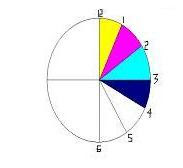Solution:
= 4π / 6
= 2π / 3

Half of the circle is π. In the diagram above, the half of the clock is 6. Divide the π into 6 equal parts. We need to find the radian between the minute and hour of the 4:00. Therefore;

In 4:00 = 4π / 6 or 2π / 3

2. A bicycle wheel has a diameter of 0.4 meters. A point on the outer rim travelled 2.6 meters. How many radians has the wheel turned?

given: 0.4 diameter
0.2 radius
2.6 meters travelled

Solution:

= x / 2π = L / 2πr
= x / 2π = 2.6 / 2π(0.2)
= x / 2π = 2.6 / 0.4π
= x = 2π(2.6) / 0.4π
= x = 5.2π / 0.4π
= x = 13
which is 13 is in quadrant 1.

What we do here is we make the equation proportional. So we have x / 2π = L / 2πr (which is the circumference of the circle).

Mr. K also discuss on how to find the quadrants of a given number. For example:

1. 17 = it is located in Quadrant 4.
2. 02 = it is located in Quadrant 2.
3. 17π / 12 = it is located in Quadrant 3.

How to find these quadrants?

o Half of the circle is π which is equal to 3.14 or approximately 3. π is equivalent to 180 degrees. The whole circle which is 360 degrees or 2π is equivalent to approximately 6.

If you want to find 11:
180 degrees is equivalent to 3
360 degrees is equivalent to 6
back again; 180 degrees is equivalent to 9
360 degrees is equivalent to 12 so move down a little bit therefore 11 is in quadrant 4.

3. In a circle of radius 6 cm, a sector has a central angle of 30 degrees. What is the area of this sector in square centimeter?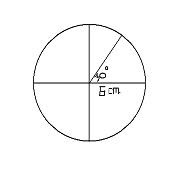Solution:

One of our classmates came up to a great answer. He makes the solution proportional. He put “A” as an area over πr^2 which is the area of the circle = 30 / 360.

= 30 / 360 = A / πr^2
= 30 / 360 = A / π(6)^2
= 30 / 360 = A / 36π
= A = 36π(30) / 360
= A = 1080π / 360
= A = 3π cm^2

We used πr^2 because we are looking for the “area” of the sector.

4. Is point (1 / square root of 5 , 2 / square root of 5) on the unit circle?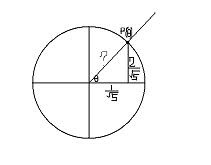Solution:

By simpy looking at the illustration the hypotenuse of the triangle must be 1.

We can used the equation of the unit circle.

= x^2 + y^2 = 1
=(1 / square root of 5)^2 + (2 / square root of 5)^2 = 1
=(1 / 5) + (4 / 5) = 1
=(5 / 5) = 1
Therefore, (1 / square root of 5 , 2 / square root of 5) is on the unit circle.

Mr. K also told us to find the sin(tetta), cos (tetta), tan (tetta) of the illustration in question #4.

We all know the magic word SOHCAHTOA.

The blog that Luis post, he explain the meaning of SOHCAHTOA and what the used of it.
Let’s review again what we had in our class about how to find sin (tetta), cos (tetta) and tan (tetta) of the question number #4.

sin (tetta) = opposite / hypotenuse
= (2 / square root of 5) / 1
= 2 square root of 5

cos (tetta) = adjacent / hypotenuse
= (1 square root of 5) / 1
= 1 square root 5

tan (tetta) = opposite / adjacent
= ( 2 square root of 5) / ( 1 square root of 5)
= 2

How about let’s try this one.
Find the sin (tetta), cos (tetta) and tan ( tetta) of:

***P(tetta) is on a ray passing through the point (6,8)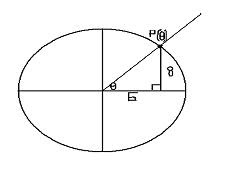In this diagram we can find the hypotenuse of this triangle by using pythagorian theorem.

= c^2 = a^2 + b^2
= c^2 = (6)^2 + (8)^2
= c^2 = 36 +84
= c^2 = 100
= c = 10
therefore the hypotenuse is 10.

sin (tetta) = opposite / hypotenuse
= 8 / 10
= 4 / 5

cos (tetta) = adjacent / hypotenuse
= 6 / 10
= 3 / 5

tan (tetta) = opposite / adjacent
= 8 / 6
= 4 / 3

I hope you learn something new this day, like I am.

And tomorrow make sure to asked Mr. K for the story of the guy who died because of triangles.

The next scribe is Jordan.

### Today's Slides: September 10

Here they are ...

To see a larger image of the slides go here. When you get there you'll see a button in the bottom right-hand corner that says [full]. Click it and the slides will display in full screen mode.

## Sunday, September 9, 2007

### Students Made This

Blogging is a very public activity. Anything that gets posted on the internet stays there. Forever. Deleting a post simply removes it from the blog it was posted to. Copies of the post may exist scattered all over the internet. I have come across posts from my students on blogs as far away as Sweden! That is why we are being so careful to respect your privacy and using first names only. We do not use pictures of ourselves. If you really want a graphic image associated with your posting use an avatar -- a picture of something that represents you but IS NOT of you.

Here are a few videos that illustrate some of what I want you to think about:

Two teachers in the U.S.A. worked with their classes to come up with a list of guidelines for student bloggers.

One of them, Bud Hunt, has these suggestions, among others:

1. Students using blogs are expected to treat blogspaces as classroom spaces. Speech that is inappropriate for class is not appropriate for our blog. While we encourage you to engage in debate and conversation with other bloggers, we also expect that you will conduct yourself in a manner reflective of a representative of this school.

2. Never EVER EVER give out or record personal information on our blog. Our blog exists as a public space on the Internet. Don’t share anything that you don’t want the world to know. For your safety, be careful what you say, too. Don’t give out your phone number or home address. This is particularly important to remember if you have a personal online journal or blog elsewhere.

3. Again, your blog is a public space. And if you put it on the Internet, odds are really good that it will stay on the Internet. Always. That means ten years from now when you are looking for a job, it might be possible for an employer to discover some really hateful and immature things you said when you were younger and more prone to foolish things. Be sure that anything you write you are proud of. It can come back to haunt you if you don’t.

4. Never link to something you haven’t read. While it isn’t your job to police the Internet, when you link to something, you should make sure it is something that you really want to be associated with. If a link contains material that might be creepy or make some people uncomfortable, you should probably try a different source.

Another teacher, Steve Lazar, developed a set of guidelines in consultation with his students. You can read them here.

Look over the guidelines and add the ones you like in the comments section below this post; either from one of Steve's students or one of your own. I think Bud's suggestions are excellent. We'll be using the one's I highlighted above as a basis for how we will use our blog.

Cheers,
Mr. K.

### Unit Circle

Hello guys, this is Luis posting. Mr. K started the class by giving us some advice/tips on how to properly use the internet as well as internet etiquette and I myself was really amazed on what I had heard. We discussed the homework assignment that he gave us regarding Degree and Radian, converting Degree and Radian and vice versa. Some questions in the assignment were just a review that we encountered in Pre-cal 11. Most of us struggled to solve the questions in the assignment because we almost forgot how to do it.

You can quickly look at the problems on the above slides and these are their brief explanations. If you have any questions on how we came up with the answer, feel free to ask me or Mr. K.

Oliver has already posted the conversion of Degrees to Radian and vice versa and this first slide is just another example of it.

The 3rd slide is question number 5 Exercise 1 on our assignment, we all know that π is equal to 180. So, if the measure of the whole triangle is equal to 180 it therefore equals to π. Mr. K. told us that we can solve this through mental math. We just have to add the two given angles and subtract it from π.

On the 4th and 5th slides, he showed us the easier way to solve parallel lines intersected by a transversal. In his example the corresponding angles have the same size and shape therefore those angles are equal. We used the formula D/2π=R/2πr.

He also gave a brief recap of what we had learned in Pre-Cal 11 such as Laws of Exponents, simplifying radicals and using quadratic formula.

On the 13th slide Mr. K. gave us a problem to solve and one of our classmates came up with the correct answering using the formula S= θr. Although, she came up with the correct answer, S= θr can only be used in one circumstance which may be the reason why Mr. K doesn’t teach this formula. The formula D/360=L/2πr can be used in many places, though it’s a long process it is more concise and more proportional.

On the other hand, if the given is asking for Radian, instead of using D/360=L/2πr we can use the 2π which is equal to 360 to express the answer. The formula will be D/2π=L/2πr.

For example:
Determine the length of the arc of the circle with the radius 8 cm that subtends each angle at the centre. Express each length to one decimal place if necessary.

1. 30°

Given:
Radius= 8
Degree = 30°

D/360=L/2πr
30°/360=L/2π(8)

0 = 30°(16π)/360
0 = 4.2°

On the 18th to 20th slides, we had used the SOHCAHTOA which means:

SOH used for sin which is sin θ= opposite / hypotenuse
CAH used for cos which is cos θ = adjacent / hypotenuse and
TOA used for tangent θ = opposite / adjacentIn this triangle the hypotenuse is 40. Why 40? Because the hypotenuse will always be twice the size of the opposite side, and the opposite side will always be half the size of hypotenuse.

To find the adjacent, we can use the cosine rule or the Pythagorean Theorem.

a^2+b^2 = c^2
b^2 = c^2 – a^2
= 40^2 – 20^2
= root of 1200
= 20 root of 3

On the 20th slide, they are all corresponding angles because they are all equal to 30° even though they are on the different quadrants. So, basically 150° is the same as 30°. The Quadrant I and the Quadrant IV are positive because the adjacent of the two angles are on the positive side of the x-axis. However, the II and III quadrants are both negative because two adjacent are on the negative side of the x-axis.

On the 21st slide, he talked about the reference angle. On his example, sine 300 and sine -60 is both equal to -0.8666. The reference angle of 300 is 60.

On the last slide, he then had given us a short recap on how to simplify radicals.

The next scribe is Precious.

## Friday, September 7, 2007

### Today's Slides: September 7

Here they are ...

To see a larger image of the slides go here. When you get there you'll see a button in the bottom right-hand corner that says [full]. Click it and the slides will display in full screen mode.

## Thursday, September 6, 2007

### Assignments #1 and #2

Some people needed copies of tonight's homework. Here are the questions and solutions from the first two homework exercises ...

Exercise 1 Questions: Page 1 of 2, Page 2 of 2

Exercise 1 Solutions: Page 1 of 2, Page 2 of 2

Exercise 2 Questions: Page 1 of 2, Page 2 of 2

Exercise 2 Solutions: Page 1 of 5, Page 2 of 5, Page 3 of 5, Page 4 of 5, Page 5 of 5

### FIRST SCRIBE POST ! " UNIT CIRCLE "

ALRIGHT-Y FOLKS ! Sorry about having the post really late, but I hope there isn't an exact deadline yet for posting the blogs ! Anyways, today's math class all began with Mr. K finishing off where he had started from yesterday, and quickly finished off with the slides he was showing to the class about being in class on time and habits, and etc. Now, this is the time where Mr. K pops up a question which leads into what we learned today, the question was:

Why are there 360 degrees in a circle?

Now, I know what you're thinking, you're probably going " EHHHH !?? " I know I did, because Mr. K surprises you =P.

Some people must have had answers but were sh
y to shout it out, but I myself was not able to come up with an answer to my head, however there was this one answers which was quite interesting, and the person had commented that it was related to the calendar and made a very interesting comparison, however it was so interesting I can't explain it myself, but I knew what he said. Unfortunately that wasn't the answer Mr. K was looking for, but it was a very good answer props to that guy, it blew my own mind away. Now, back to the topic about calendars, Mr. K mentioned an interesting fact about how the deck of cards represents a calendar, that was cool.

Getting back to the question
Why are there 360 degrees in a circle? Mr. K led us to the answer by asking us if 360 was divisible by all the numbers we could think of. We came up with

1,2,3,4,5,6,8,9,10,13,15,20,24,30,36,44,45,60,72,90,
120,180,360. These are a lot of numbers ! So therefore, from that, the answer was, that 360 is the number of degrees in a circle because it cut into evenly pieces.

Mr. K had then given us some math questions to do.
1. x/4.5 = 7/9
2. 4/x = 13/
π
3.
π/8 = x/180
4. 31/50 = π/x

To make life easier, here are the answers: *REMEMBER, IF ANSWERS ARE IN THE DECIMALS, THEY SHOULD BE IN THE 4th PLACE, AND HE HAS HEARD YOU SAY THAT YOU HAVE ALL HEARD IT, DO NOT FORGET! =).

1. x/4.5 = 7/9
x = 4.5(7)/9
x = 3.5

2. 4/x = 13/π
x = 4π /13
x = 0.9666

3. π/8 = x/180
x = 180π/8
x = 70.6858

4. 31/50 = π/x
x = 50π/31
x = 5.0671

Those are all the answers for the questions that were in red. Also, these questions, do not need to be answered in decimal approximation, but rather in an "exact fraction."

For example, 4/x = 13/
π => 4π/13

Later on, Mr. K introduced the class to the "Unit Circle"That is the unit circle filled with all the radian measures around the circle, looks confusing, but it isn't really, if you try to look at it closer ! Mr. K will teach us more about it in later classes, or better yet, ask him about it on your free time, and also on his free time, for better understanding of the unit circle, and DON'T BE SCARED ! Or else ! you'll never learn, if you don't ask QUESTIONS !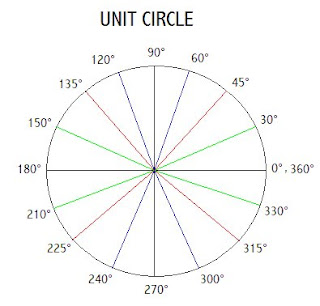This diagram is of the Unit Circle has measurements in degrees.

Shortly after Mr. K introduced this he then gave us the question:
Convert 20° into radians.

20
°/180° = θ/π
= 20
π = 180(θ) = 20π/180 = θ
= π/9 = θ

Then
he also showed us how to convert radians into degrees.
Convert
π/5 into degrees.

θ/180 = π/5 / π ( this is called " complex fraction )
= θ/180 = (π/5)(1/π)
= 5
θ = 180
=
θ = 180/5
=
θ = 36°

We basically, learned how to convert degrees and radians
, this is the equation given at the end of class.

D(angle in degrees) / 180
° = R(angle in radians) / π

Alas, this is at the end of my scribe post, and I hope you've all enjoyed reading, and study hard everyone. Before I forget, these are the two Phrases you'll hear a lot during this semester of Mr. K's math class.

"Fractions are our friends" "Mathematics is a science of patterns"

remember them well, and you'll DO well in the class, and of course asking questions and studying and forming groups with people you do not know ;).

TUNE IN FOR NEXT CLASS ! A story of a guy who died because of a triangle, dun dun dunnnnn ......

### Today's Slides: Sept. 6

Here they are ...

To see a larger image of the slides go here. When you get there you'll see a button in the bottom right-hand corner that says [full]. Click it and the slides will display in full screen mode.

## Wednesday, September 5, 2007

### A New Beginning ...

Hi There! You found our blog! This is the place to talk about what's happening in class; to ask a question you didn't get a chance to ask in class; to get copies of a handout you didn't get in class (here's the course outline); for parents to find out "How Was School Today;" to share your knowledge with other students. Most importantly it's a place to reflect on what we're learning.

Remember what I said about the Forgetting Curve? Well a big part of Learning and Remembering involves working with and discussing new ideas with other people -- THIS is the place to do just that. Use the comment feature below each post, or make your own post, contribute to the conversation and lets get down to some serious blogging!

Here are the slides from today (your homework is on slide #25):

See you tomorrow.

Cheers,
Mr. K.